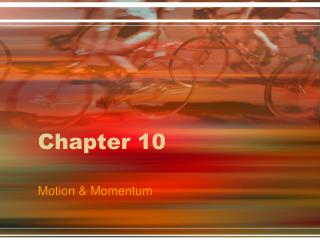# Chapter 10 - PowerPoint PPT PresentationDownload PresentationChapter 10

Download Presentation## Chapter 10

- - - - - - - - - - - - - - - - - - - - - - - - - - - E N D - - - - - - - - - - - - - - - - - - - - - - - - - - -
##### Presentation Transcript

1. Chapter 10 Motion & Momentum

2. Ch 10.1 – What is Motion? • All matter is constantly in motion • Motion involves change in position 1. An object changes position relative to a reference point

3. 2. Distance – the total length of the route an object travels when it moves

4. 3. Displacement – includes the distance and direction of the stopping point from the starting point

5. C. Speed – distance traveled divided by the time taken to travel that distance 1. Formula for speed: speed = distance/time 2. SI Unit for speed: meters per second (m/s)

6. Calculating Speed • Calculate the speed of a swimmer who swims 100m in 56s • Speed = distance / time or • D = 100m • T = 56s • Speed = 100m = 1.8 m/s 56s

7. 3. An object in motion can change speeds many times as it moves from one point to another, speeding up or slowing down a) Average speed – the total distance traveled divided by total time taken

8. b) Instantaneous speed – an object’s speed at a particular moment in time c) Constant speed – occurs when an object travels at a steady rate with the same instantaneous speed for some period of time

9. D. Graphing Motion 1. Motion can be graphed on a distance-time graph a) Distance is plotted on the vertical axis b) Time is plotted on the horizontal axis

10. 2. The steeper the line the faster the speed

11. 3. A horizontal line indicates that no change in position is occurring and the speed is zero

12. E. Velocity – the speed of an object AND its direction of motion 1. Velocity changes if either or both of these factors is altered

13. Ch 10.2 - Acceleration • Acceleration – change in velocity divided by the time for the change to occur 1. Can include an object speeding up, slowing down, and/or changing direction

14. B. Acceleration can be calculated if you know how an object’s velocity has changed during a given time period 1. Formula for acceleration: Acceleration = final speed – initial speed time a = (Sf – Si) / t

15. a) SI Unit for acceleration: meters per second squared (m/s2) b) Acceleration number will be positive when an object is speeding up and negative when slowing down

16. Calculating Acceleration • Calculate the acceleration of a bus whose speed changes from 6m/s to 12m/s over a period of 3s • A = (final speed – initial speed) time • A = 12 m/s – 6 m/s = 6 m/s = 2 m = 2 m/s2 3 s 3 s s x s

17. C. Graphing Acceleration 1. Acceleration can be graphed with a speed – time graph a) Speed is plotted on the vertical axis b) Time is plotted on the horizontal axis

18. 2. An object that is speeding up will have a line that slopes upward 3. An object that is slowing down will have a line that slopes downward 4. An object moving at a constant speed will have an acceleration of zero, and a horizontal line on the graph

19. Speed – Time Graph AKA… (speed) (constant speed) Positive Acceleration Negative Acceleration

20. Ch 10.3 - Momentum • The amount of matter that an object has is its mass 1. Inertia – the tendency of an object to resist change in motion; directly correlates to its mass

21. B. Momentum – measure of how hard it is to stop an object 1. Calculated as mass times velocity 2. Formula for momentum: momentum = mass x velocity (p = mv)

22. Calculating Momentum • Calculate the momentum of a 14-kg bicycle traveling north at 2 m/s • P = mv • P = 14 kg x 2 m/s north • P = 28 kg x m/s north

23. Which has more Momentum?

24. 4. Momentum has direction that is the same direction as its velocity ex: P = 28 kg x m/s north

25. C. Law of Conservation of Momentum 1. The total momentum of objects that collide with each other does not change

26. 2. There are many ways collisions can occur a) Objects may stick together and move still stuck together b) Objects may bounce off each other and transfer momentum from one to the other

27. 3. In all cases, the total momentum of the objects that collide is the same before and after the collision